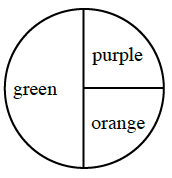Home > CC1MN > Chapter cc21 > Lesson cc21.2.7 > Problem1-124

1-124.

What is the probability of getting either blue or green on a spinner that is $\frac { 3 } { 10 }$ green and $\frac { 1 } { 5 }$ blue? Show your work. Homework Help ✎Refer to the Math Notes in Lesson 1.2.8 to change the fraction of blue parts to an equivalent fraction so that both fractions have the same denominator.

Add both fractions to get the probability of getting blue or green on the spinner.